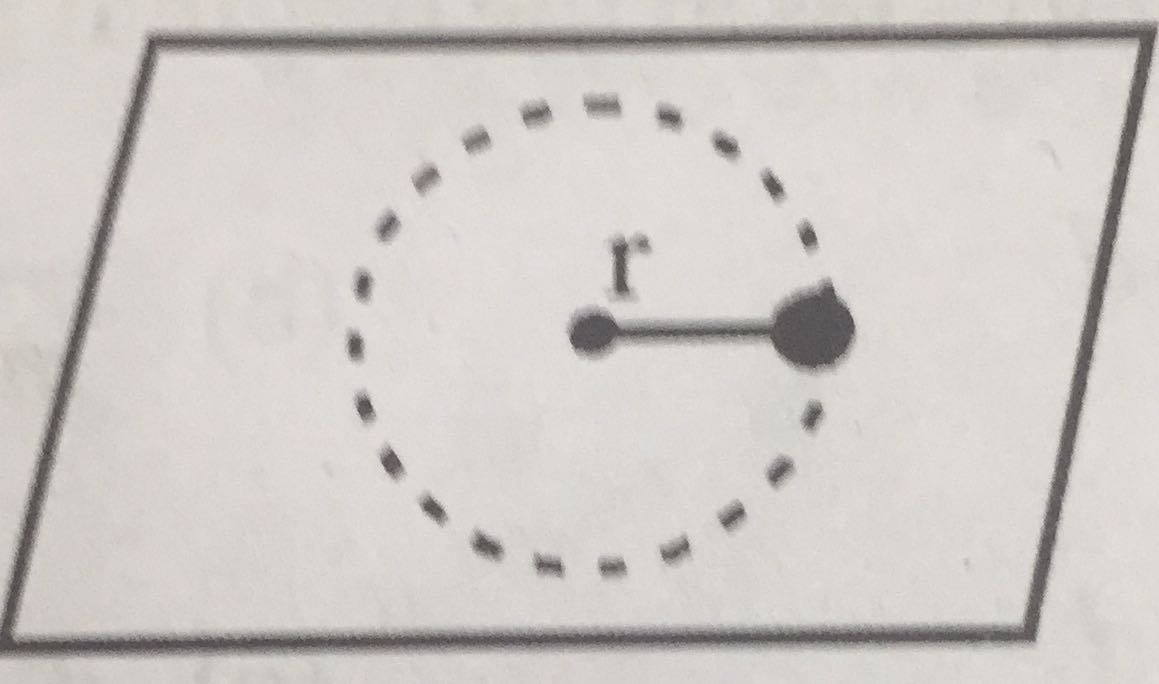# A small mass attached to a string rotates on frictionless table top as shown. If the tension in the string is increased by pulling the string causing the radius of the cutcular motion to decrease by a factor of 2, the kinetic eneegy of the mass willOption 1) remain constant Option 2) increase by a factor of 2 Option 3) increase by a factor of 4 Option 4) decrease by a factor of 2

V Vakul

As discussed in

Angular momentum -

- wherein

represent angular momentum of a moving particle about a point.

it can be calculated  as

= Length of perpendicular on line of motion

= component of momentum along perpendicualar to

is increased by a factor of 4

Option 1)

remain constant

This option is incorrect.

Option 2)

increase by a factor of 2

This option is incorrect.

Option 3)

increase by a factor of 4

This option is correct.

Option 4)

decrease by a factor of 2

This option is incorrect.

Exams
Articles
Questions